# Potential outcomes, counterfactuals, causal effects, and randomization

Next week I’ll be attending the third UK Causal Inference Meeting, in Bristol. Causal inference has seen a tremendous amount of methodological development over the last 20 years, and recently a number of books have been published on the topic. In advance of attending the conference, I’ve been reading through a draft of the excellent book by Miguel Hernán (who is giving a pre-conference course) and James Robins on ‘Causal Inference’ (freely downloadable here). So far I’ve found the book highly readable and intuitive. As I’m working through it, I thought I’d write some posts giving overviews of some of the material covered, which I personally find useful to help cement the ideas in my own mind, and possibly might be of use to others.

Potential outcomes and counterfactuals
The first chapter of their book covers the definition of potential outcomes (counterfactuals), individual causal effects, and average causal effects. As Hernán and Robins point out right at the start of their book, we all have a good intuitive sense of what it means to say that an intervention A causes B. By far the most popular approach to mathematically defining a causal effect is based on potential outcomes, or counterfactuals. For simplicity, we consider an intervention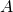, which is either absent, as indicated by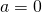, or present, indicated by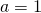. We consider a single binary outcome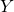, which takes values 0 or 1.

In order to define what we mean by a causal effect, for each individual (or subject, or unit) we assume the existence of the potential outcomes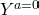and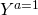, corresponding to what value the outcome would take if we did not apply the intervention (i.e.) or did apply the intervention () respectively. For an individual, we say that the intervention has a causal effect whenever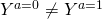, that is the outcome would take a different value depending on whether the individual is given the intervention or not.

To calculate the causal effect of the intervention on a given individual we would need to somehow obtain or discover the valuesand. However, we immediately face a fundamental difficulty: we cannot simultaneously observe both values. Imagine that we perform a study, and through some mechanism some individuals receive the intervention and others do not. We letdenote a random variable indicating whether an individual receives the intervention (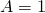) or not (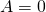), anda random variable for the observed outcome. If a particular individual received the intervention, their observed value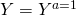(assuming we make the so called consistency assumption). But for this individual the potential outcome valueis unknown. Consequently, in most situations we cannot determine the causal effect at the level of an individual.

Average causal effects
If we cannot estimate individual causal effects, what can we do? One possible easier target is a so called average causal effect. The averaging here corresponds to averaging the (unobservable) individual causal effects across the individuals in some well defined population. Imagine for a moment that all individuals in the population were not given the intervention. The mean of their outcomes in this situation is simply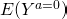, i.e. the average of the potential outcomes whenis set for all individuals. Similarly,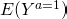is the population average of the potential outcomes if all individuals received the intervention. The (or rather a) average causal effect is then defined as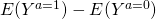, that is the difference between these two quantities.

Under certain assumptions, it is possible to estimate such average causal effects. They have an obvious and clear usefulness in regards to whether giving an intervention to a population will have an effect the outcome of interest, and if so, how large this effect will be. The above definition of the average causal effect is given as the difference in the mean potential outcomes. As Hernán and Robins explain, there are many other possible quantities which could be used to define a population causal effect, for example, in the case of a binary outcome, the risk ratio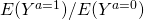.

An interesting point to note is that it is possible for a population average causal effect to be zero even though some individual causal effects are non-zero. This can occur because the non-zero individual cause effects of different individuals could (in principle) cancel each other out, such that the overall average causal effect is zero.

Estimation of causal effects through randomized experiments
Having defined individual and population average causal effects, the next logical step is to ask under what conditions can we estimate the latter, and how can we do so. One way is to perform a randomized experiment or trial, in which the decision as to whether each individual receives the intervention or not. If the intervention allocation is randomized, the mean of the observed outcomes in those individuals randomized to receive the treatment,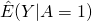is an unbiased estimate of.

One way of seeing why this is the case is to make the connection with missing data. Specifically, as noted earlier, for each individual we only get to observe on of their two potential outcome values, which one depending on whether they received the intervention or not. When we calculate the mean of the outcomes in those allocated to receive the intervention, we are performing a complete case analysis, withbeing the indicator that someone is a complete case for the potential outcome under intervention. The key is that when we use randomization, the allocation variableis statistically independent of any other variable. In particular, this means thatis independent of the potential outcome(the value thattakes when the individual receives the intervention). Because of randomization, the missing values ofare missing completely at random. Consequently, the complete case mean amongst those allocated to receive the intervention is unbiased for the population mean. The same is true for those allocated not to receive the intervention, which gives us an estimate of, and then we can simply calculate the difference: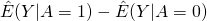to estimate the average causal effect,.

This site uses Akismet to reduce spam. Learn how your comment data is processed.